Search by Topic

Resources tagged with Addition & subtraction similar to Diagonal in a Spiral:

Filter by: Content type:
Age range:
Challenge level:

There are 211 results

Broad Topics > Calculations and Numerical Methods > Addition & subtractionDiagonal in a Spiral

Age 7 to 11 Challenge Level:

Investigate the totals you get when adding numbers on the diagonal of this pattern in threes.Sheep Talk

Age 7 to 11 Challenge Level:

In sheep talk the only letters used are B and A. A sequence of words is formed by following certain rules. What do you notice when you count the letters in each word?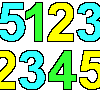Sept03 Sept03 Sept03

Age 7 to 11 Challenge Level:

This number has 903 digits. What is the sum of all 903 digits?Age 5 to 11 Challenge Level:

Try out this number trick. What happens with different starting numbers? What do you notice?Zios and Zepts

Age 7 to 11 Challenge Level:

On the planet Vuv there are two sorts of creatures. The Zios have 3 legs and the Zepts have 7 legs. The great planetary explorer Nico counted 52 legs. How many Zios and how many Zepts were there?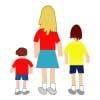Sisters and Brothers

Age 7 to 11 Challenge Level:

Cassandra, David and Lachlan are brothers and sisters. They range in age between 1 year and 14 years. Can you figure out their exact ages from the clues?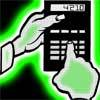Reverse Trick

Age 7 to 11 Challenge Level:

Tell your friends that you have a strange calculator that turns numbers backwards. What secret number do you have to enter to make 141 414 turn around?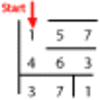Maze 100

Age 7 to 11 Challenge Level:

Can you go through this maze so that the numbers you pass add to exactly 100?The Clockmaker's Birthday Cake

Age 7 to 11 Challenge Level:

The clockmaker's wife cut up his birthday cake to look like a clock face. Can you work out who received each piece?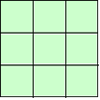1, 2, 3 Magic Square

Age 7 to 11 Challenge Level:

Arrange three 1s, three 2s and three 3s in this square so that every row, column and diagonal adds to the same total.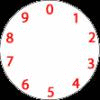Dividing a Cake

Age 7 to 11 Challenge Level:

Annie cut this numbered cake into 3 pieces with 3 cuts so that the numbers on each piece added to the same total. Where were the cuts and what fraction of the whole cake was each piece?The Deca Tree

Age 7 to 11 Challenge Level:

Find out what a Deca Tree is and then work out how many leaves there will be after the woodcutter has cut off a trunk, a branch, a twig and a leaf.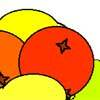Oranges and Lemons

Age 7 to 11 Challenge Level:

On the table there is a pile of oranges and lemons that weighs exactly one kilogram. Using the information, can you work out how many lemons there are?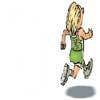Rocco's Race

Age 7 to 11 Short Challenge Level:

Rocco ran in a 200 m race for his class. Use the information to find out how many runners there were in the race and what Rocco's finishing position was.Calendar Calculations

Age 7 to 11 Challenge Level:

Try adding together the dates of all the days in one week. Now multiply the first date by 7 and add 21. Can you explain what happens?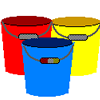Buckets of Thinking

Age 7 to 11 Challenge Level:

There are three buckets each of which holds a maximum of 5 litres. Use the clues to work out how much liquid there is in each bucket.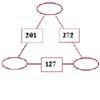Number Juggle

Age 7 to 11 Challenge Level:

Fill in the missing numbers so that adding each pair of corner numbers gives you the number between them (in the box).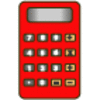Clever Keys

Age 7 to 11 Short Challenge Level:

On a calculator, make 15 by using only the 2 key and any of the four operations keys. How many ways can you find to do it?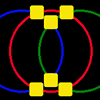Magic Circles

Age 7 to 11 Challenge Level:

Put the numbers 1, 2, 3, 4, 5, 6 into the squares so that the numbers on each circle add up to the same amount. Can you find the rule for giving another set of six numbers?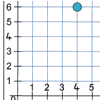Two Number Lines

Age 7 to 11 Challenge Level:

Max and Mandy put their number lines together to make a graph. How far had each of them moved along and up from 0 to get the counter to the place marked?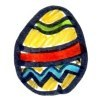How Many Eggs?

Age 7 to 11 Challenge Level:

Peter, Melanie, Amil and Jack received a total of 38 chocolate eggs. Use the information to work out how many eggs each person had.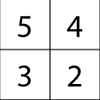Magic Matrix

Age 7 to 11 Challenge Level:

Find out why these matrices are magic. Can you work out how they were made? Can you make your own Magic Matrix?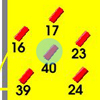Throw a 100

Age 7 to 11 Challenge Level:

Can you score 100 by throwing rings on this board? Is there more than way to do it?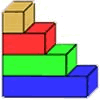Rod Measures

Age 7 to 11 Challenge Level:

Using 3 rods of integer lengths, none longer than 10 units and not using any rod more than once, you can measure all the lengths in whole units from 1 to 10 units. How many ways can you do this?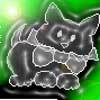Christmas Shopping

Age 7 to 11 Challenge Level:

Vera is shopping at a market with these coins in her purse. Which things could she give exactly the right amount for?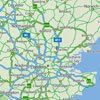Lengthy Journeys

Age 7 to 11 Challenge Level:

Investigate the different distances of these car journeys and find out how long they take.Bundles of Cubes

Age 7 to 11 Challenge Level:

Watch this animation. What do you notice? What happens when you try more or fewer cubes in a bundle?Journeys

Age 7 to 11 Challenge Level:

Investigate the different distances of these car journeys and find out how long they take.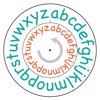Some Secret Codes

Age 7 to 11 Challenge Level:

Can you follow the rule to decode the messages?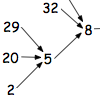Age 7 to 11 Challenge Level:

What happens when you add the digits of a number then multiply the result by 2 and you keep doing this? You could try for different numbers and different rules.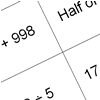How Do You Do It?

Age 7 to 11 Challenge Level:

This group activity will encourage you to share calculation strategies and to think about which strategy might be the most efficient.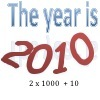It Was 2010!

Age 5 to 11 Challenge Level:

If the answer's 2010, what could the question be?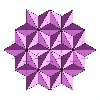Next Number

Age 7 to 11 Short Challenge Level:

Find the next number in this pattern: 3, 7, 19, 55 ...Being Resilient - Primary Number

Age 5 to 11 Challenge Level:

Number problems at primary level that may require resilience.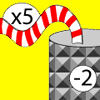Function Machines

Age 7 to 11 Challenge Level:

If the numbers 5, 7 and 4 go into this function machine, what numbers will come out?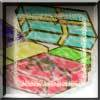Age 7 to 11 Challenge Level:

Use the information to work out how many gifts there are in each pile.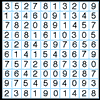A Numbered Route

Age 7 to 11 Challenge Level:

Can you draw a continuous line through 16 numbers on this grid so that the total of the numbers you pass through is as high as possible?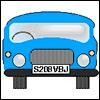Numbered Cars

Age 7 to 11 Challenge Level:

I was looking at the number plate of a car parked outside. Using my special code S208VBJ adds to 65. Can you crack my code and use it to find out what both of these number plates add up to?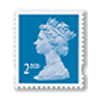Penta Post

Age 7 to 11 Challenge Level:

Here are the prices for 1st and 2nd class mail within the UK. You have an unlimited number of each of these stamps. Which stamps would you need to post a parcel weighing 825g?Napier's Bones

Age 7 to 11 Challenge Level:

The Scot, John Napier, invented these strips about 400 years ago to help calculate multiplication and division. Can you work out how to use Napier's bones to find the answer to these multiplications?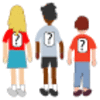One Wasn't Square

Age 7 to 11 Challenge Level:

Mrs Morgan, the class's teacher, pinned numbers onto the backs of three children. Use the information to find out what the three numbers were.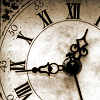Clock Face

Age 7 to 11 Challenge Level:

Where can you draw a line on a clock face so that the numbers on both sides have the same total?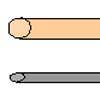A Rod and a Pole

Age 7 to 11 Challenge Level:

A lady has a steel rod and a wooden pole and she knows the length of each. How can she measure out an 8 unit piece of pole?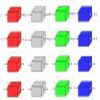Machines

Age 7 to 11 Challenge Level:

What is happening at each box in these machines?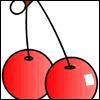Cherries Come in Twos

Age 7 to 11 Challenge Level:

Susie took cherries out of a bowl by following a certain pattern. How many cherries had there been in the bowl to start with if she was left with 14 single ones?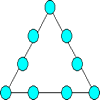Magic Triangle

Age 7 to 11 Challenge Level:

Place the digits 1 to 9 into the circles so that each side of the triangle adds to the same total.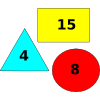Super Value Shapes

Age 7 to 11 Challenge Level:

If each of these three shapes has a value, can you find the totals of the combinations? Perhaps you can use the shapes to make the given totals?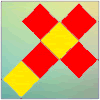Let's Face It

Age 7 to 11 Challenge Level:

In this problem you have to place four by four magic squares on the faces of a cube so that along each edge of the cube the numbers match.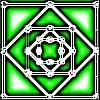Number Squares

Age 5 to 11 Challenge Level:

Start with four numbers at the corners of a square and put the total of two corners in the middle of that side. Keep going... Can you estimate what the size of the last four numbers will be?Sam's Quick Sum

Age 7 to 11 Challenge Level:

What is the sum of all the three digit whole numbers?